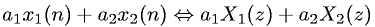Equations > Signal Processing > Z Transform Properties > Z-transform linearity property

### Z-transform linearity propertyLatex Code:

MathML Code:

 ${a}_{1}{x}_{1}\left(n\right)+{a}_{2}{x}_{2}\left(n\right)⇔{a}_{1}{X}_{1}\left(z\right)+{a}_{2}{X}_{2}\left(z\right)$

MathType 5.0: